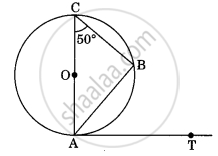# If figure AB is a chord of the circle and AOC is its diameter such that ∠ACB = 50°. If AT is the tangent to the circle at point A, the ∠BAT is equal to ______ - Mathematics

MCQ
Fill in the Blanks

If figure AB is a chord of the circle and AOC is its diameter such that ∠ACB = 50°. If AT is the tangent to the circle at point A, the ∠BAT is equal to ______• 65°

• 60°

• 50°

• 40°

#### Solution

If figure. AB is a chord of the circle and AOC is its diameter such that ∠ACB = 50°. If AT is the tangent to the circle at point A, the ∠BAT is equal to 50°.Explanation:

In figure, AOC is a diameter of the circle. We know that, diameter subtends an angle 90° at the circle.

So, ∠ABC = 90°

In ΔACB, ∠A + ∠B + ∠C = 180°  .....[Since, sum of all angles of a triangle is 180°]

⇒ ∠A + 90° + 50° = 180°

⇒ ∠A + 140° = 180°

⇒ ∠A = 180° – 140° = 40°

∠A or ∠OAB = 40°

Now, AT is the tangent to the circle at point A, OA is perpendicular to AT.

∴ ∠OAT = 90°   ......[From figure]

⇒ ∠OAB + ∠BAT = 90°

On putting ∠OAB = 40°, we get

⇒ ∠BAT = 90° – 40° = 50°

Hence, the value of ∠BAT = 50°.

Concept: Tangent to a Circle
Is there an error in this question or solution?

#### APPEARS IN

NCERT Mathematics Exemplar Class 10
Chapter 9 Circles
Exercise 9.1 | Q 3 | Page 102
Share